# Principal Component Analysis PCA BCH 364 C394 P

• Slides: 24Principal Component Analysis (PCA) BCH 364 C/394 P Systems Biology / Bioinformatics Edward Marcotte, Univ of Texas at AustinWhat is Principal Component Analysis? What does it do? So, first let’s build some intuition. “You do not really understand something unless you can explain it to your grandmother”, Albert Einstein wikipedia With thanks for many of these explanations to http: //stats. stackexchange. com/questions/2691/makingsense-of-principal-component-analysis-eigenvectors-eigenvaluesWhat is Principal Component Analysis? What does it do? A general (and imprecise) political example: Suppose you conduct a political poll with 30 questions, each answered by 1 (strongly disagree) through 5 (strongly agree). Your data is the answers to these questions from many people, so it’s 30 -dimensional, and you want to understand what the major trends are. You run PCA and discover 90% of your variance comes from one direction, corresponding not to a single question, but to a specific weighted combination of questions. This new hybrid axis corresponds to the political left-right spectrum, i. e. democrat/republican spectrum. Now, you can study that, or factor it out & look at the remaining more subtle aspects of the data. So, PCA is a method for discovering the major trends in data, simplifying the data to focus only on those trends, or removing those trends to focus on the remaining information. Example: Christian Bueno, http: //stats. stackexchange. com/questions/2691/making-sense-of-principal-component-analysis-eigenvectors-eigenvalues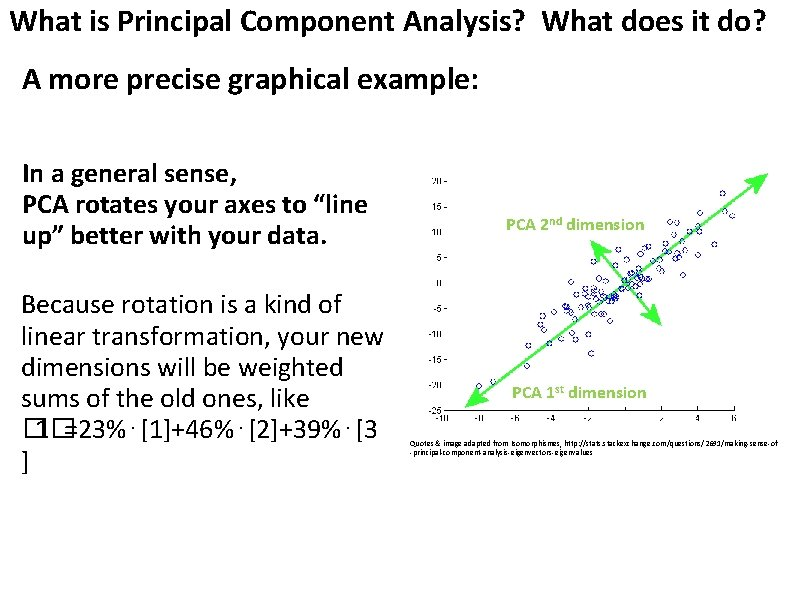What is Principal Component Analysis? What does it do? A more precise graphical example: In a general sense, PCA rotates your axes to “line up” better with your data. Because rotation is a kind of linear transformation, your new dimensions will be weighted sums of the old ones, like � 1�=23%⋅+46%⋅+39%⋅[3 ] PCA 2 nd dimension PCA 1 st dimension Quotes & image adapted from Isomorphismes, http: //stats. stackexchange. com/questions/2691/making-sense-of -principal-component-analysis-eigenvectors-eigenvalues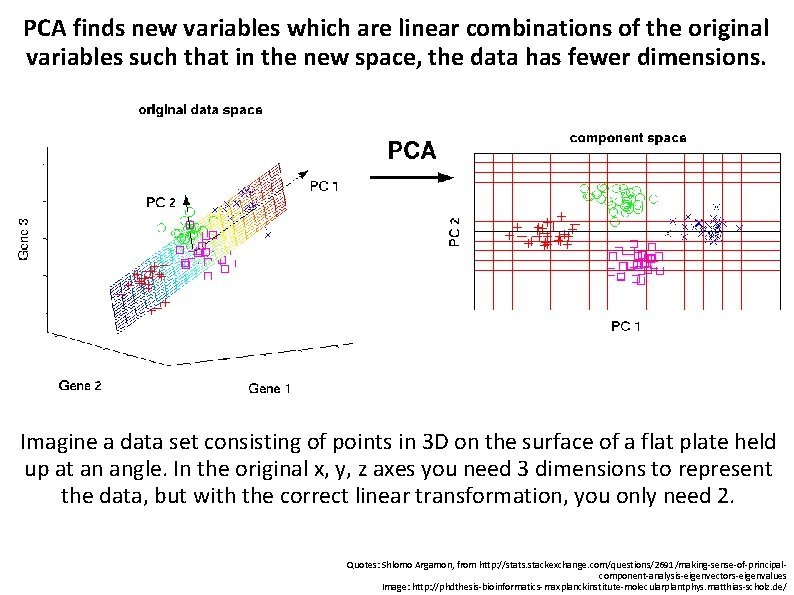PCA finds new variables which are linear combinations of the original variables such that in the new space, the data has fewer dimensions. Imagine a data set consisting of points in 3 D on the surface of a flat plate held up at an angle. In the original x, y, z axes you need 3 dimensions to represent the data, but with the correct linear transformation, you only need 2. Quotes: Shlomo Argamon, from http: //stats. stackexchange. com/questions/2691/making-sense-of-principalcomponent-analysis-eigenvectors-eigenvalues Image: http: //phdthesis-bioinformatics-maxplanckinstitute-molecularplantphys. matthias-scholz. de/To summarize so far: PCA is a technique to reduce dimension by: 1. Taking linear combinations of the original variables. 2. Each linear combination explains the most variance in the data it can. 3. Each linear combination is uncorrelated (orthogonal) with the others 4. Plot the data in terms of only the most important (principal) dimensions Image: http: //blog. equametrics. com/2013/02/an-introduction-to-principal-component-analysis Quote: http: //stats. stackexchange. com/questions/2691/making-sense-of-principal-component-analysis-eigenvectors-eigenvalues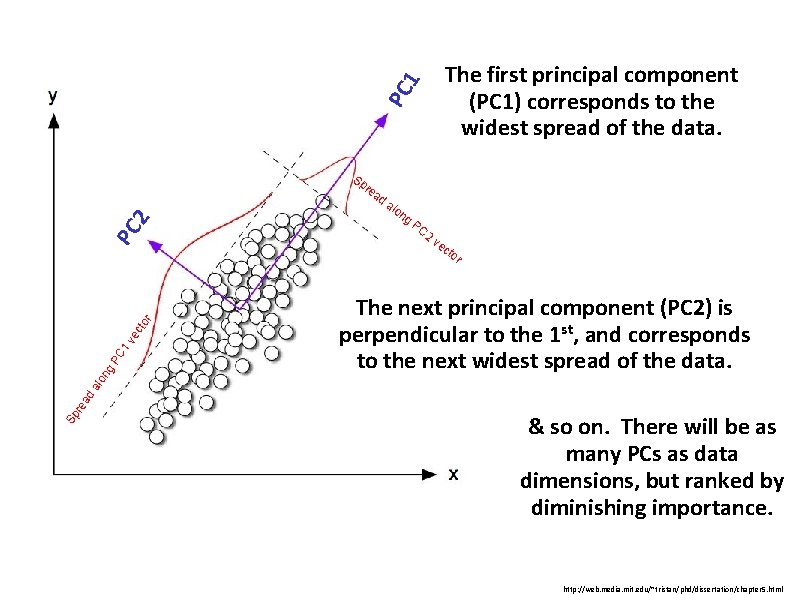PC 1 The first principal component (PC 1) corresponds to the widest spread of the data. PC 2 Sp re ad alo ng PC 2 ve cto The next principal component (PC 2) is perpendicular to the 1 st, and corresponds to the next widest spread of the data. Sp re ad alo ng PC 1 ve cto r r & so on. There will be as many PCs as data dimensions, but ranked by diminishing importance. http: //web. media. mit. edu/~tristan/phd/dissertation/chapter 5. htmlLinear Transformations Scaling x 2 XX X X XX a 2 x 2 OO O O OO OO OO x 1 O OOOO O O X X X X X a 1 x 1 Adapted from http: //www. engr. sjsu. edu/~knapp/HCIRODPR/PR_Mahal/linear_tr. htm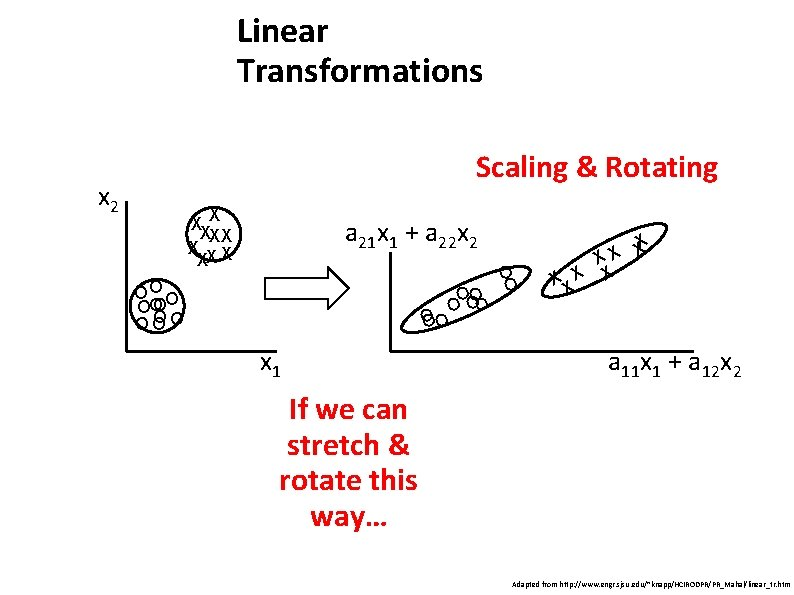Linear Transformations Scaling & Rotating x 2 XX X X XX a 21 x 1 + a 22 x 2 OO O O OO OO x 1 O O X X X X a 11 x 1 + a 12 x 2 If we can stretch & rotate this way… Adapted from http: //www. engr. sjsu. edu/~knapp/HCIRODPR/PR_Mahal/linear_tr. htm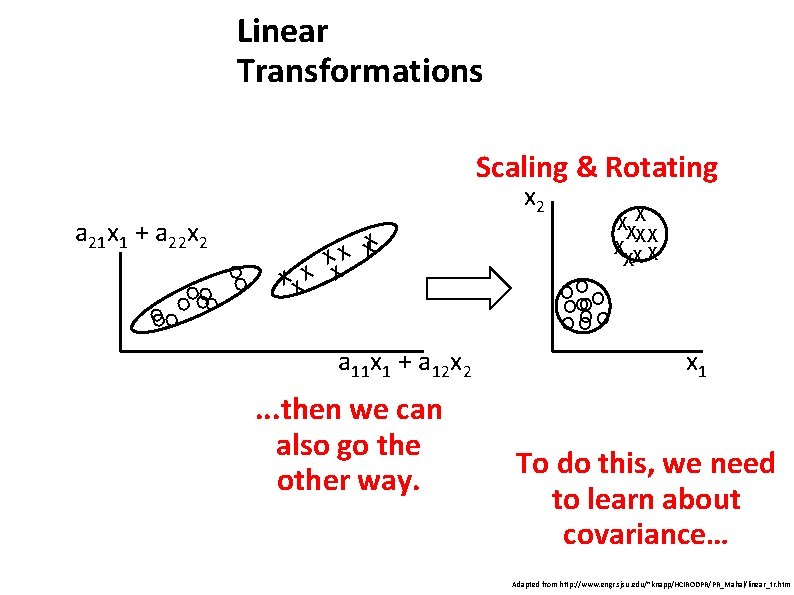Linear Transformations Scaling & Rotating x 2 a 21 x 1 + a 22 x 2 OO O O X X X X a 11 x 1 + a 12 x 2 . . . then we can also go the other way. XX X X XX OO O O OO OO OO x 1 To do this, we need to learn about covariance… Adapted from http: //www. engr. sjsu. edu/~knapp/HCIRODPR/PR_Mahal/linear_tr. htmMore examples of different linear transformations: http: //isomorphismes. tumblr. com/post/11407834056/matrices-linear-algebra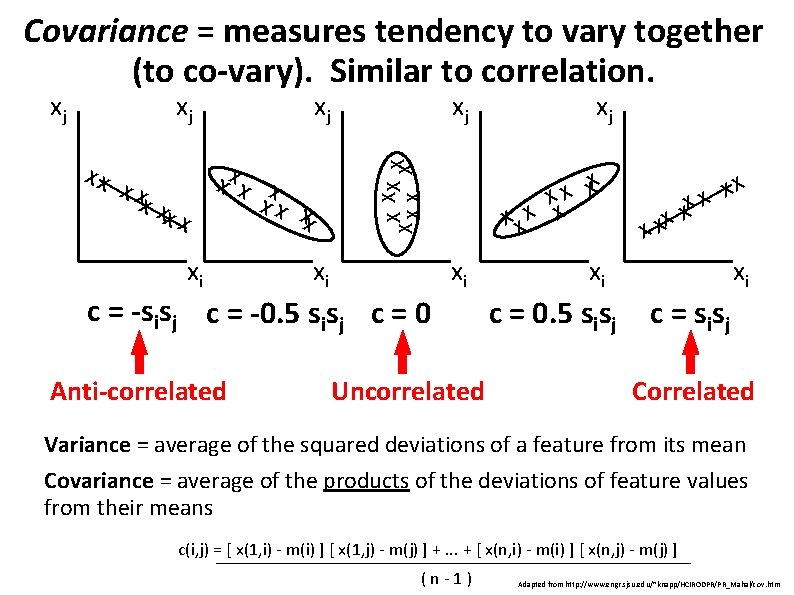Covariance = measures tendency to vary together (to co-vary). Similar to correlation. XX X X XX xi xi xj X XX X X XX xj X X XX X xj X X X xj c = -sisj c = -0. 5 sisj c = 0 Anti-correlated xi Uncorrelated xj X X X X XX xi xi c = 0. 5 sisj c = s is j Correlated Variance = average of the squared deviations of a feature from its mean Covariance = average of the products of the deviations of feature values from their means c(i, j) = [ x(1, i) - m(i) ] [ x(1, j) - m(j) ] +. . . + [ x(n, i) - m(i) ] [ x(n, j) - m(j) ] (n-1) Adapted from http: //www. engr. sjsu. edu/~knapp/HCIRODPR/PR_Mahal/cov. htm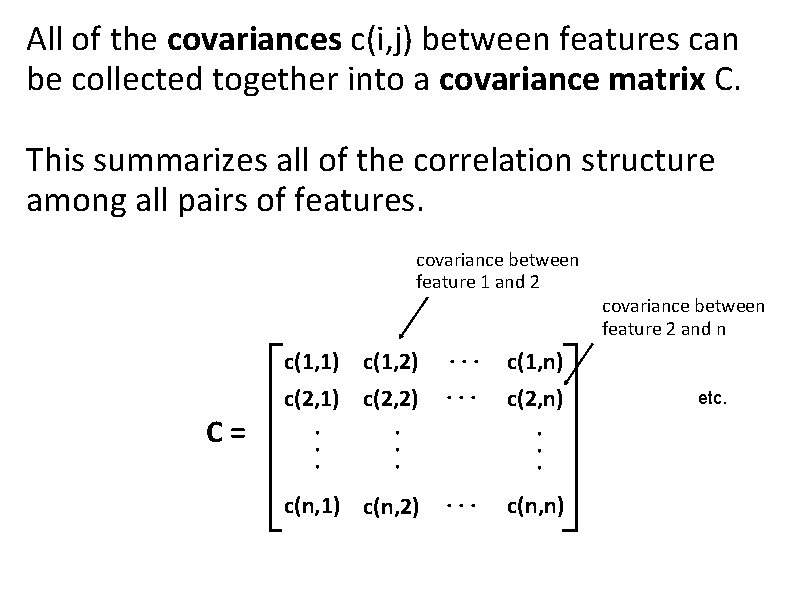All of the covariances c(i, j) between features can be collected together into a covariance matrix C. This summarizes all of the correlation structure among all pairs of features. covariance between feature 1 and 2 c(1, 1) c(1, 2) c(1, n) . . . c(n, n) c(n, 1) c(n, 2) c(2, n). . C= c(2, 1) c(2, 2) . . . covariance between feature 2 and n etc.We need one last concept: Eigenvectors and eigenvalues The blue arrow is an eigenvector of this linear transformation matrix, since it doesn’t change direction. Its scale is also unchanged, so its eigenvalue is 1. Wikipedia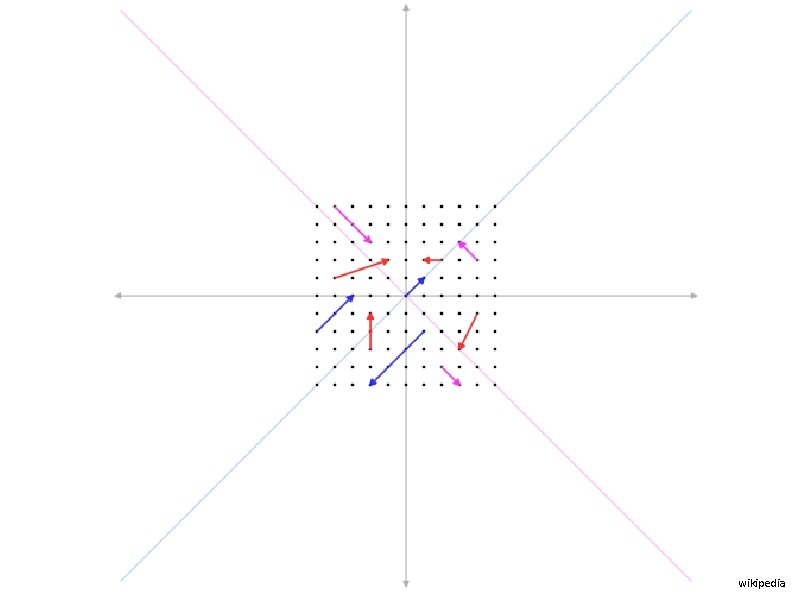wikipedia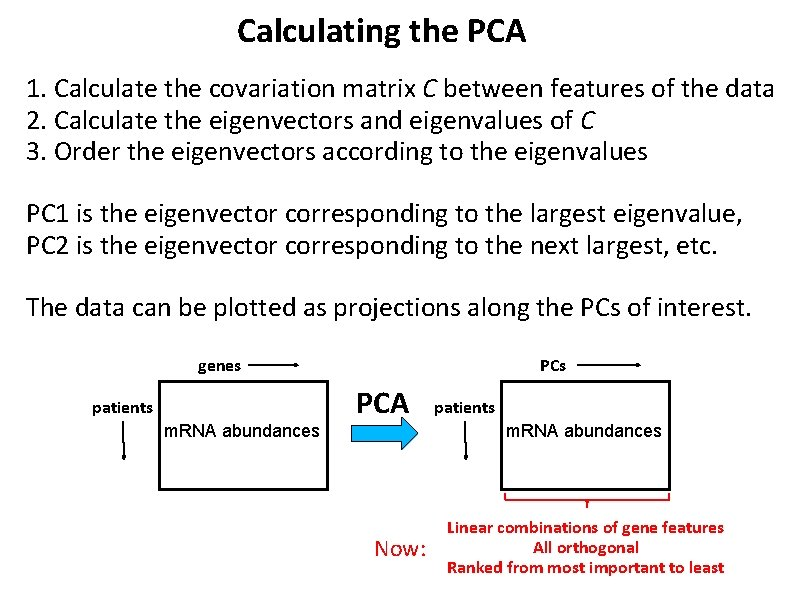Calculating the PCA 1. Calculate the covariation matrix C between features of the data 2. Calculate the eigenvectors and eigenvalues of C 3. Order the eigenvectors according to the eigenvalues PC 1 is the eigenvector corresponding to the largest eigenvalue, PC 2 is the eigenvector corresponding to the next largest, etc. The data can be plotted as projections along the PCs of interest. genes patients m. RNA abundances PCA Now: patients m. RNA abundances Linear combinations of gene features All orthogonal Ranked from most important to least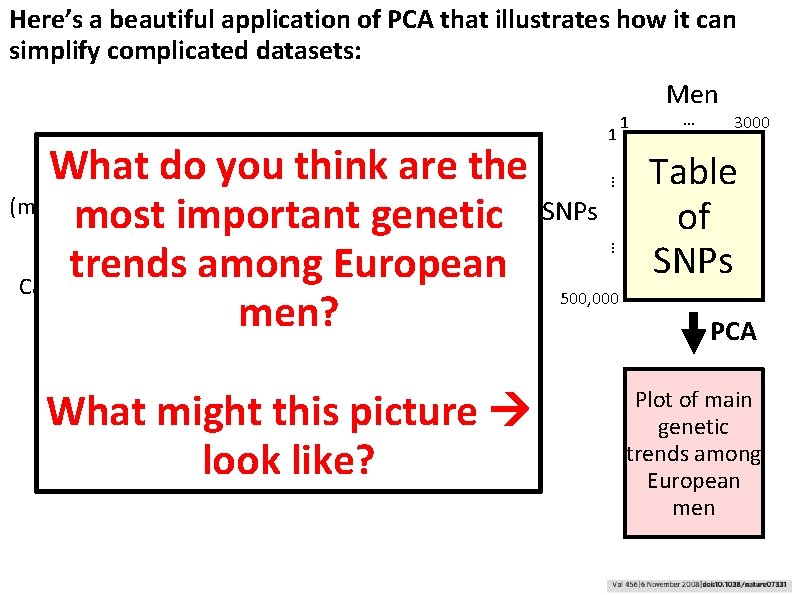Here’s a beautiful application of PCA that illustrates how it can simplify complicated datasets: Visualize their genetic relationships by plotting each man as a point in “genotype space”, emphasizing only the most dominant genetic trends What might this picture look like? … SNPs … What do~3, 000 you. European thinkmenare the Genotype (measure ~500 K SNPs each using DNA microarrays) most important genetic trends among European Calculate the most important “trends” in the data using Principal Component men? Analysis 1 1 Men … 3000 Table of SNPs 500, 000 PCA Plot of main genetic trends among European men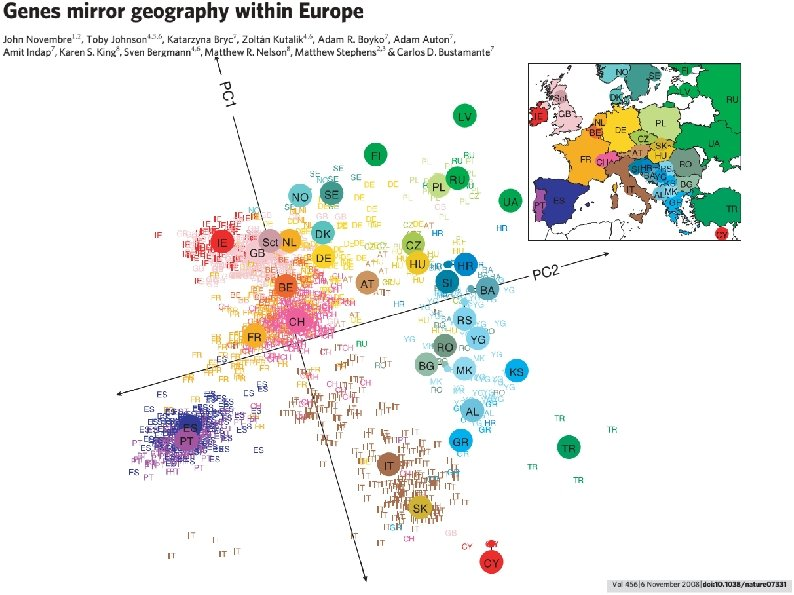This is a map of their DNA. It recapitulates the map of Europe. In other words, the strongest genetic trends across European men relate directly to their geographic locations (note: not nationality)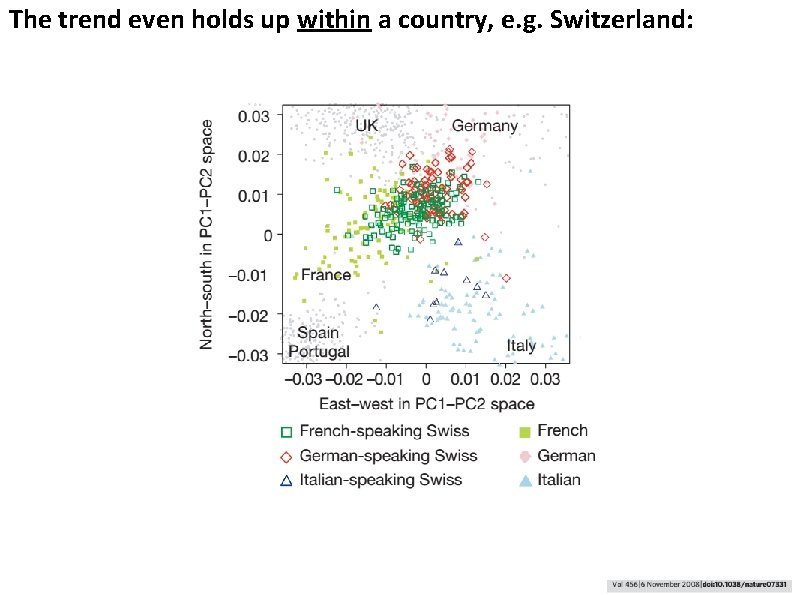The trend even holds up within a country, e. g. Switzerland:Why? Genetic similarity is strongly distance dependent in Europe, presumably because people tend to live near where they were born and tend to marry locally.The trend is so strong that it can be used to predict where the men are from, e. g. shown here by leave-one-out cross-validation:SUMMARY In a sense, PCA fits a (multidimensional) ellipsoid to the data • Described by directions and lengths of principal (semi-)axes, e. g. the axis of a cigar or egg or the plane of a pancake encyclopedia 2. thefreedictionary. com • No matter how an ellipsoid is turned, the eigenvectors point in those principal directions. The eigenvalues give the lengths. • The biggest eigenvalues correspond to the fattest directions (having the most data variance). The smallest eigenvalues correspond to the thinnest directions (least data variance). • Ignoring the smallest directions (i. e. , collapsing them) loses relatively little information. Adapted from whuber, http: //stats. stackexchange. com/questions/2691/making-sense-of-principal-component-analysis-eigenvectors-eigenvalues